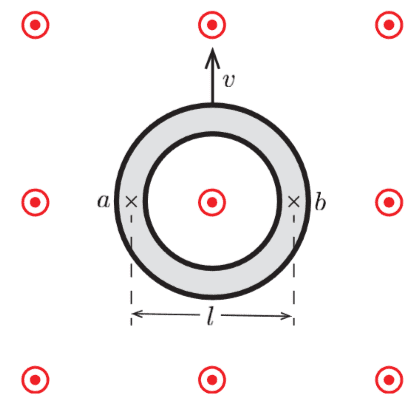# Hall effect over a conducting ring

Trisztan
Homework Statement:
What is the voltage around a conducting ring of diameter ##l##, moving through a uniform magnetic field of magnitude ##B##, at speed ##v##?
Relevant Equations:
Definition of potential difference:
$$\Delta V = -\int_i^f {\mathbf{E}\cdot \mathrm{d}\mathbf{s}}$$
Force magnitude equivalence under the Hall effect:
$$qE = qvB$$
This is the diagram provided in the question:The ring is made of conducting material. I was originally asked to find the potential difference between ##a## and ##b##. I did so using the Hall effect (and assuming it would work as per normal in this situation). This got me ##\Delta V = vBl##.

However, I am now lost on how to find the "voltage around the ring". If I had to guess, I would say its 0 everywhere around the ring except at either ##a## and ##b## (depending on which you take to be the point where ##V=0##).

Any help would be much appreciated.

Homework Helper
Gold Member
You need to do a line integral $$\int_a^b \mathbf{E}\cdot d\mathbf{s}=\int_a^b (\mathbf{v}\times \mathbf{B})\cdot d\mathbf{s}$$ where ##d\mathbf{s}## is a line element along the circular path.

Trisztan
@kuruman Sorry, but I don't understand how that will help me. Wouldn't that just give me ##-\Delta V## between ##a## and ##b##? I already have that, don't I?

Homework Helper
Gold Member
It will give you the integral around the semicircle which is what (I think) the question is asking you to find. You wrote that you didn't know how to do it and I explained that to you. Maybe someone else has another answer that is more to your liking. Stick around.

BTW what you call the Hall effect is more often called "motional emf". Here is a brief description of the Hall effect.

•berkeman
Trisztan
@kuruman No need to get so defensive man, your answer was perfectly to my liking. I simply didn't understand exactly what you were saying.

But I see now why you want to take the line integral around the semi-circle; that should probably be it. Thank you.

And yeah the link you mentioned is what I was referring to.